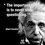# complex trigonometry??

Prove that -$\frac{i}{2}$Ln($\frac{a+ix}{a-ix}$) = arctan($\frac{x}{a}$)

i = $\sqrt{-1}$

Post the solution if you have solved itNote by Abdulmuttalib Lokhandwala
7 years, 2 months ago

This discussion board is a place to discuss our Daily Challenges and the math and science related to those challenges. Explanations are more than just a solution — they should explain the steps and thinking strategies that you used to obtain the solution. Comments should further the discussion of math and science.

When posting on Brilliant:

• Use the emojis to react to an explanation, whether you're congratulating a job well done , or just really confused .
• Ask specific questions about the challenge or the steps in somebody's explanation. Well-posed questions can add a lot to the discussion, but posting "I don't understand!" doesn't help anyone.
• Try to contribute something new to the discussion, whether it is an extension, generalization or other idea related to the challenge.

MarkdownAppears as
*italics* or _italics_ italics
**bold** or __bold__ bold
- bulleted- list
• bulleted
• list
1. numbered2. list
1. numbered
2. list
Note: you must add a full line of space before and after lists for them to show up correctly
paragraph 1paragraph 2

paragraph 1

paragraph 2

[example link](https://brilliant.org)example link
> This is a quote
This is a quote
    # I indented these lines
# 4 spaces, and now they show
# up as a code block.

print "hello world"
# I indented these lines
# 4 spaces, and now they show
# up as a code block.

print "hello world"
MathAppears as
Remember to wrap math in $$ ... $$ or $ ... $ to ensure proper formatting.
2 \times 3 $2 \times 3$
2^{34} $2^{34}$
a_{i-1} $a_{i-1}$
\frac{2}{3} $\frac{2}{3}$
\sqrt{2} $\sqrt{2}$
\sum_{i=1}^3 $\sum_{i=1}^3$
\sin \theta $\sin \theta$
\boxed{123} $\boxed{123}$

Sort by:

Differentiate both the sides individually. Doing that shall yield $\frac{a}{a^{2}+x^{2}}$ on both sides,

- 7 years, 2 months ago

Note that you still have to show that they are equal at one point, otherwise the graphs could be vertical shifts of each other.

Don't forget your constant $+ C$ when integrating!

Staff - 7 years, 2 months ago

Yes sir I have taken care of the constant c while integrating the function in two ways and they are equal at one point.

- 7 years, 2 months ago

$The\quad exponential\quad form\quad of\quad Tan(z)\quad is\\ i\frac { { e }^{ -iz }-{ e }^{ iz } }{ { e }^{ -iz }+{ e }^{ iz } } \\ If\quad z=-\frac { i }{ 2 } Log(\frac { a+ix }{ a-ix } ),\quad then\quad Tan(z)\quad is\\ i({ (\frac { a+ix }{ a-ix } ) }^{ -\frac { 1 }{ 2 } }-{ (\frac { a+ix }{ a-ix } ) }^{ \frac { 1 }{ 2 } })({ (\frac { a+ix }{ a-ix } ) }^{ -\frac { 1 }{ 2 } }+{ (\frac { a+ix }{ a-ix } ) }^{ \frac { 1 }{ 2 } })^{ -1 }\\ Simplifying\quad this\quad reduces\quad it\quad to\quad \frac { x }{ a }$

- 7 years, 2 months ago

Thanks Michael for the solution I got these result by integrating the function $\frac{1}{x^2+a^2}$ by two different methods

- 7 years, 2 months ago

Vaidya already provided the other method, so I thought I'd include the exponential form route. You know, the brute force way.

- 7 years, 2 months ago

As I have mentioned below, it should be $\frac{a}{x^2+a^2}$ and not $\frac{1}{x^2+a^2}$

- 7 years, 2 months ago

Ya both are true but finally when you do integration a will Already get cancelled and will get the same result

- 7 years, 2 months ago

Beautiful method!!

- 7 years, 2 months ago

- 7 years, 1 month ago

nice post

- 7 years, 1 month ago